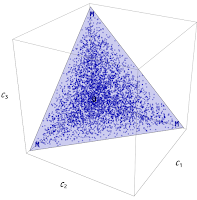## Saturday, April 29, 2017

### High dimensional budget constraints and economic growth

This is something of a partial idea, a work in progress. Let's say there is some factor of production $M$ allocated across $p$ different firms. The $p$-volume bounded by this budget constraint is:

$$V = \frac{M^{p}}{p!}$$p-volume bounded by budget constraint M

Let's say total output $N$ is proportional to the volume $V$. Take the logarithm of the volume expression

$$\log V = p \log M - \log p!$$

and use Stirling's approximation for a large number of firms:

$$\log V = p \log M - p \log p + p$$

If we assume $V \sim e^{\nu t}$ and $M \sim e^{\mu t}$ and taking the (logarithmic) derivative (continuously compounded rate of change) and re-arranging a bit:

$$\nu = \left( p+ \left( t - \frac{\log p}{\mu} \right) \frac{dp}{dt} \right) \mu$$

Now let's take $p \sim e^{\pi t}$ and re-arrange a bit more:

$$\text{(1) }\; \nu = p \left( 1 + \left(1 - \frac{\pi}{\mu} \right) \pi t \right) \mu$$

In the information equilibrium model, for exponential growing functions with growth rates $a$ and $b$ we have the relationship (see e.g. here)

$$a = k b$$

where $k$ is the information transfer index. So in equation (1) we can identify the IT index

$$k \equiv p \left( 1 + \left(1 - \frac{\pi}{\mu} \right) \pi t \right)$$

In a sense, we have shown one way how the information equilibrium condition with $k \neq 1$ can manifest itself. For short time scales $\pi t \ll 1$, we can say $p \approx p_{0} (1 + \pi t)$ and:

$$k \approx p_{0} \left( 1 + \pi t + \left(1 - \frac{\pi}{\mu} \right) \pi t \right)$$

This an interesting expression. If $\pi > 2 \mu$ then the IT index falls. That is to say if the rate at which the number of firms increases faster than the factor of production increase, then the IT index falls. Is this the beginning of an explanation for falling growth with regard to secular stagnation? I'm not so sure.

As I said, this is a work in progress.